# How to Extract Text before @ Sign from an Email Address in Excel?

• Last Updated : 21 Aug, 2021

You got a dataset and you want to extract the name part of the email address. This task can be done with a text to column and formula that uses the LEFT and FIND functions.

## Using LEFT and FIND functions

### The LEFT function:

The LEFT function returns a given text from the left of our text string based on the number of characters specified.

Attention reader! Don’t stop learning now. If you are an Excel beginner (or an intermediate) and want to learn Excel, Geeksforgeeks brings the perfect course for you to start, Diving Into Excel

Syntax:

`LEFT(text, [num_chars])`

Parameters:

• Text: The text we want to extract from.
• Num_chars (Optional): The number of characters you want to extract. Default num_chars is 1 and the number must be a positive number that is greater than zero.

Example: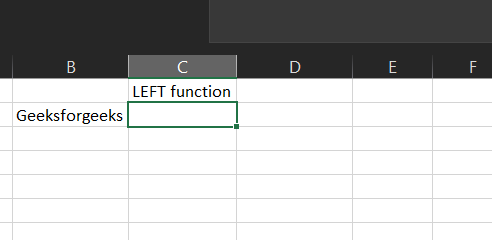Now if you want to get the first geeks from geeksforgeeks in B2. Let us follow the next step.

Step 2: We will enter =LEFT(B2,5) in the B3 cell. Here we want Excel to extract the first 5 characters from the left side of our text in B2.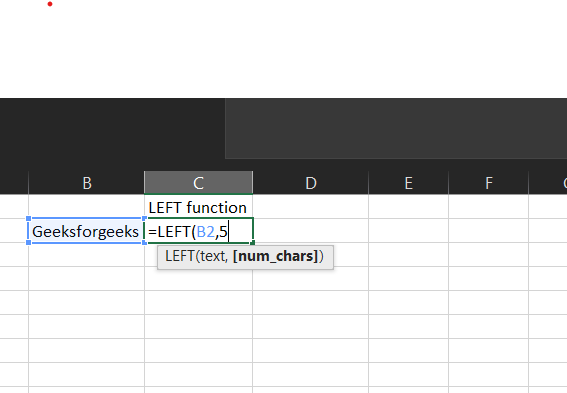This will return Geeks.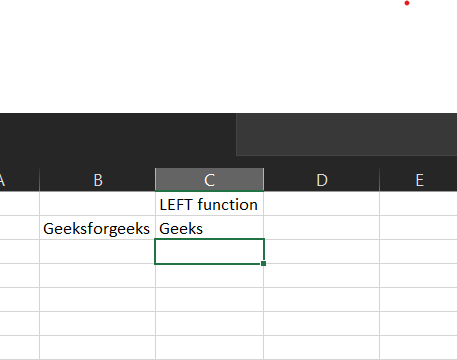Let us move to the FIND function.

### The FIND function:

The FIND function returns the position of a given text within a text.

Syntax:

`FIND(find_text, within_text, [start_num])`

Parameters:

• Find_text: The text we want to find.
• Within_text: The text containing our find_text.
• Start_num (Optional): The starting position of our find_text. Default is 1.

Example: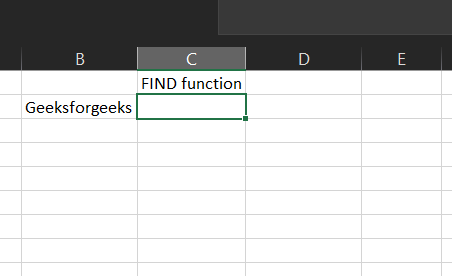Now if you want to find “for” in geeksforgeeks in B2. Let us follow the next step.

Step 2: We will enter =FIND(“for”,B2) in B3 cell. Here we want Excel to find “for” in our text in B2.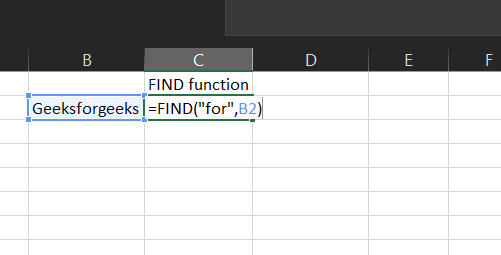This will return 6 because “for” is located at character number 6 in our text.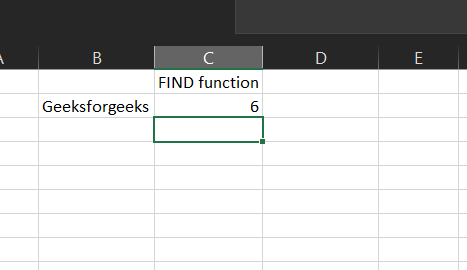Now let’s use both to extract text before the “@” in an email address.

Step 1: Format our data.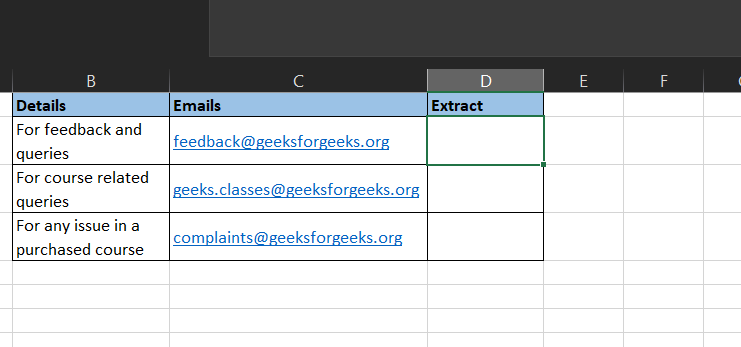Step 2: Let’s find the location of the “@” from the email. Here we use the FIND function.

We will enter =FIND(“@”,C2) in C3 cell. Here we want Excel to find “@” in our text in C2.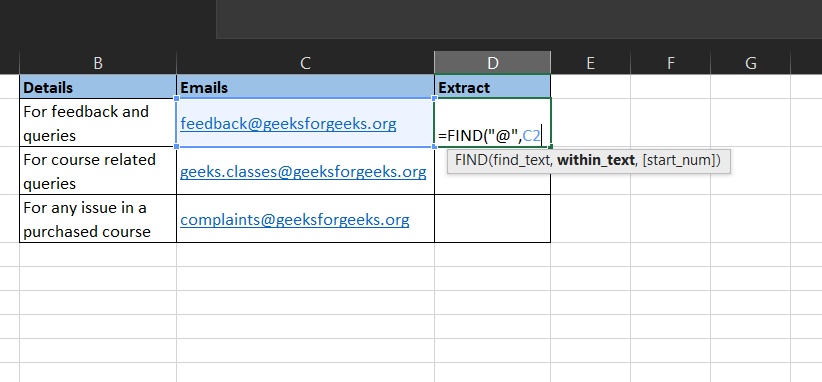Step 3: This will return 9. Next, because we want the text and not the number, we would use the LEFT function while utilizing the location function of the FIND function.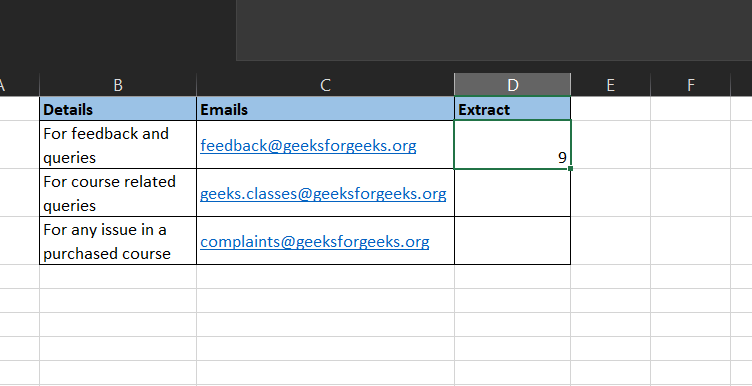Step 4:  We will enter =LEFT(C2,FIND(“@”,C2)) in the C3 cell. Here we want Excel to extract the number of characters defined by our FIND function from the left side of our text in C2.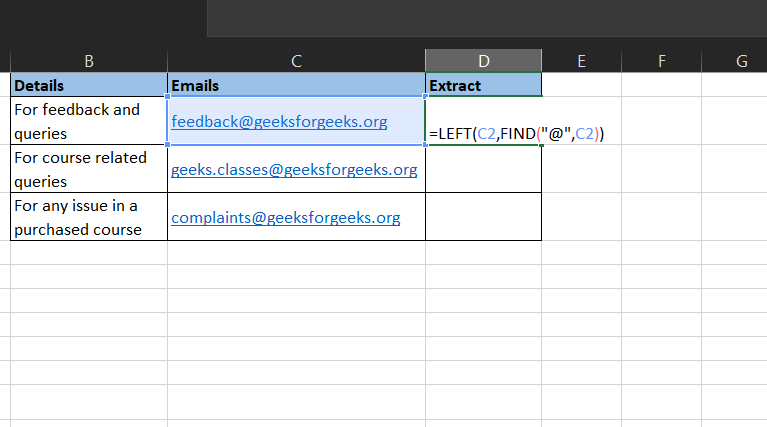Step 5: But this returns a “feedback@”.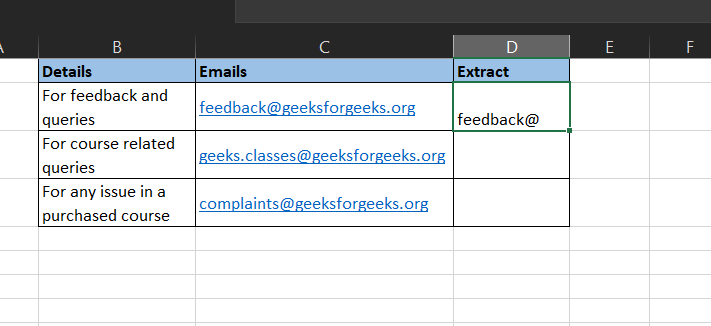Step 6: Now we need to modify the formula. Thus we add a -1 so we get the text exactly right before the “@”.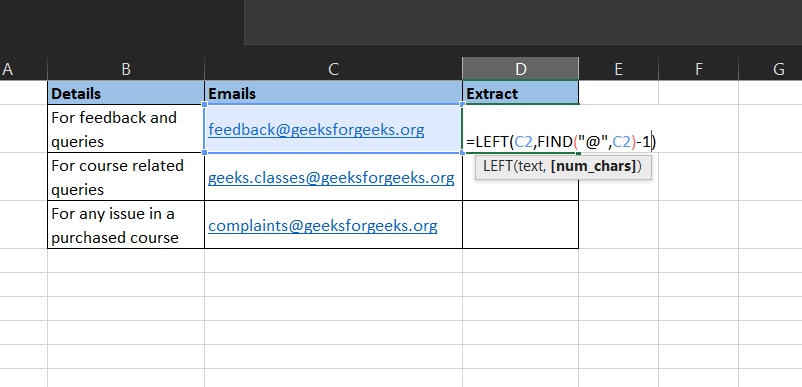Step 7: This returns what we want “feedback”. Now we can drag the formula down.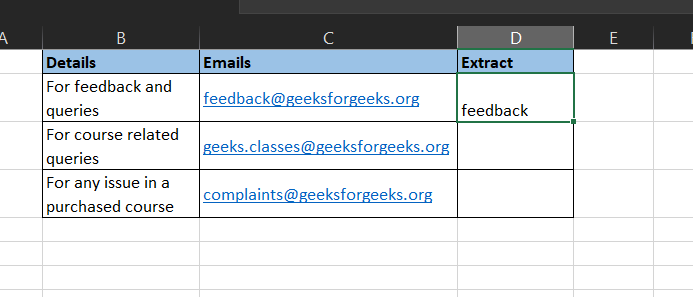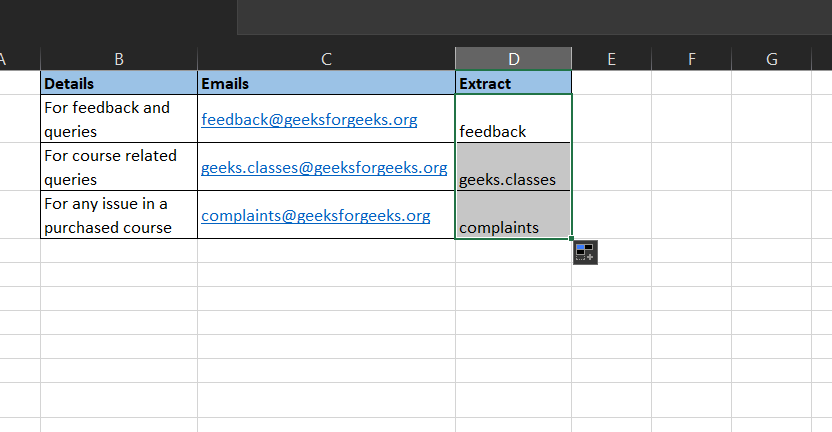## Using Text to columns

This would extract it using the delimiter aspect of the text to column features of excel:

Step 1: We format our data.Step 2: Select column C, which contains the email address.

Step 3: Move to the Data ribbon and click on Text to Columns.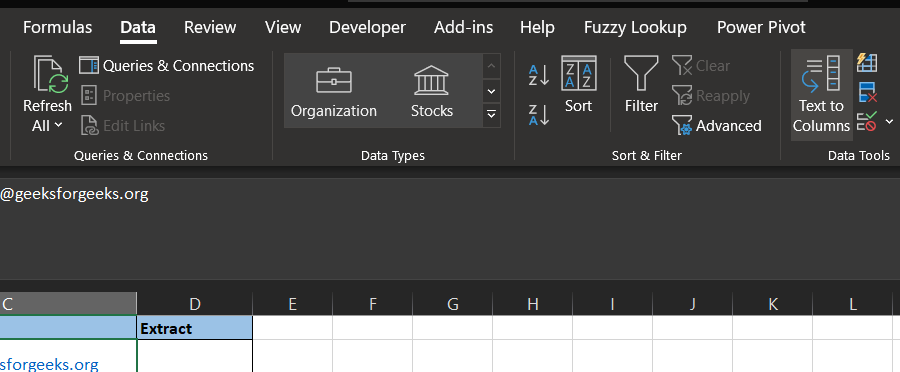Step 4: The Text to column box pops up. Though, the default is often Delimited, select Delimited if it is not. Click on Next.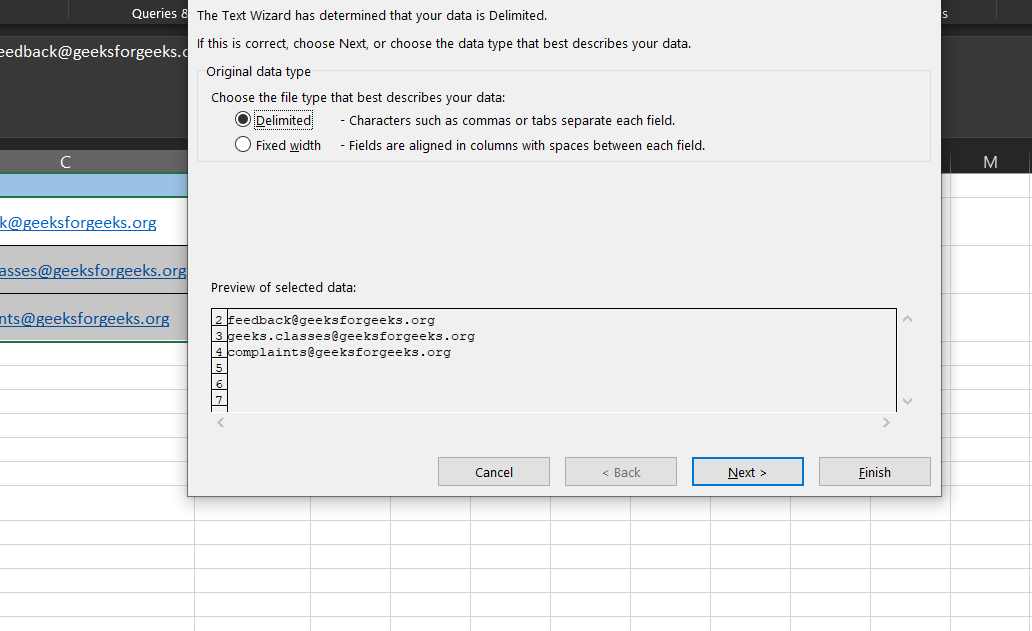Step 5: Untick the tab, tick the other check box and write down “@”. Click on Next.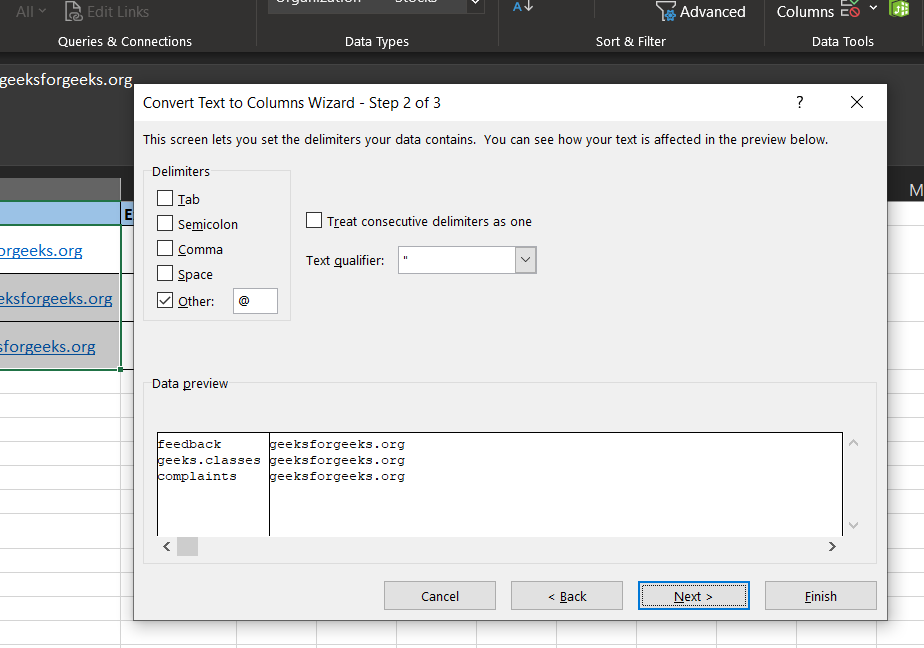Step 6: Specify your location. We would change the \$C\$2 to \$D\$2 because we want the result in cell D2. Click on Finish.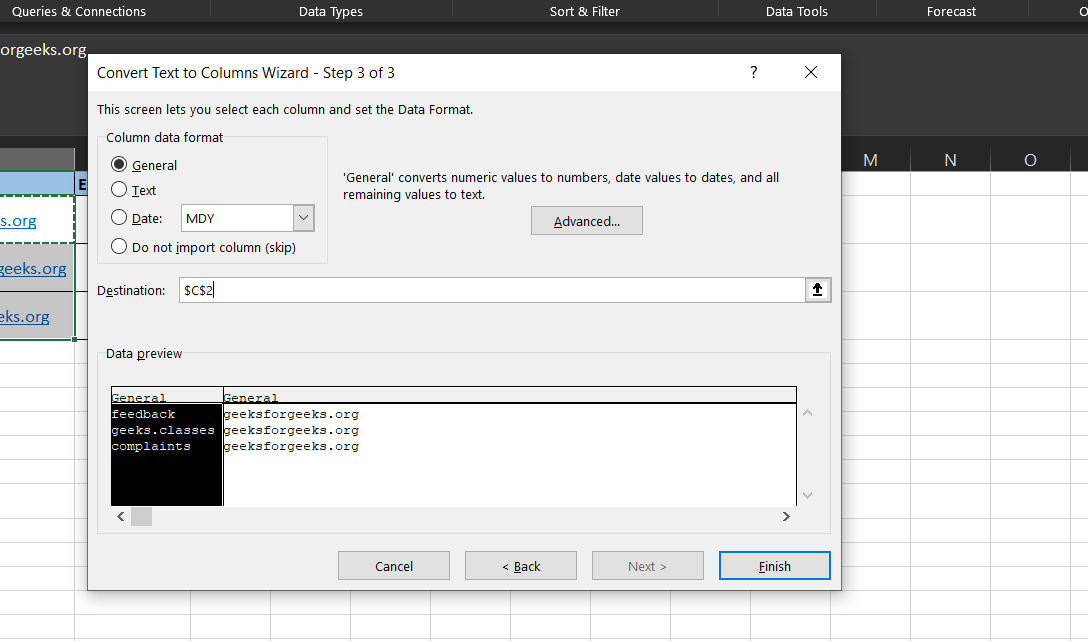Step 7: We delete the content in the E column.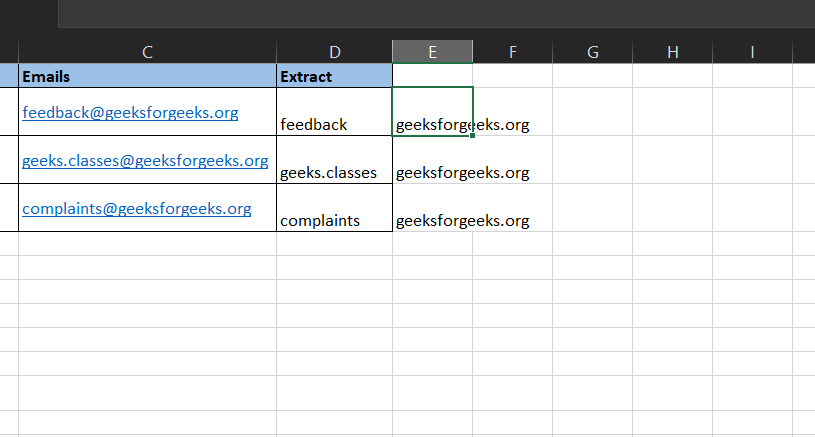Step 8: Viola! We have our text before the “@”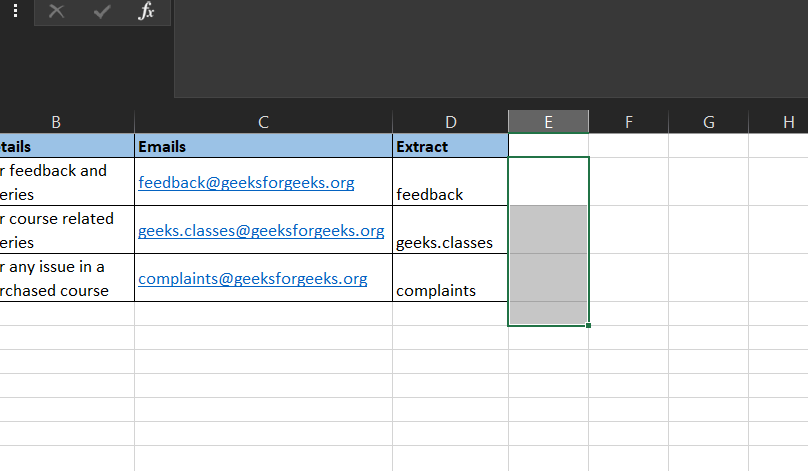My Personal Notes arrow_drop_up